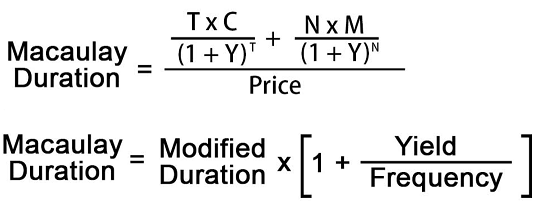#Macaulay Duration Calculator

This Frederick Macaulay calculator is used to measure of the bonds sensitivity to the changes in interest rates.

# Results...

Macaulay Duration = 3.5662

Modified Macaulay Duration = 3.4623

Number of Payments = 8

Present Value of Bond = \$103.51

Annual Coupon Payment = \$7## Formula for Macaulay Duration Calculation :

The Macaulay duration is the weighted average term to maturity of the cash flows from a bond. The weight of each cash flow is determined by dividing the present value of the cash flow by the price. This calculator uses the below formula to calculate the macaulay duration.Where,

T - Total Time Period

C - Coupon Payment

Y - Yield

N - Total Number of Periods

M - Maturity

Price - Face Value or Present Value of All the Cash Flows